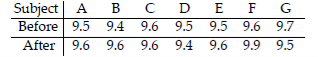• FAQ
• Contact/ Homework Answers / Statistics / Use the traditional method of hypothesis testing to test the
Not my Question
Flag Content

# Question

Use the traditional method of hypothesis testing to test the given claim about the means of two populations. Assume that two dependent samples have been randomly selected from normally distributed populations.

15) A coach uses a new technique to train gymnasts. 7 gymnasts were randomly selected and their competition scores were recorded before and after the training. The results are shown below.Using a 0.01 level of significance, test the claim that the training technique is effective in raising the gymnasts' scores.

Determine the decision criterion for rejecting the null hypothesis in the given hypothesis test; i.e., describe the values of the test statistic that would result in rejection of the null hypothesis.

16) We wish to compare the means of two populations using paired observations. Suppose that

d = 3.125, Sd = 2.911, and n = 8, and that you wish to test the following hypothesis at the 10% level of significance:

H0: μd = 0 against H1: μd > 0.

What decision rule would you use?

A) Reject H0 if test statistic is less than 1.415.

B) Reject H0 if test statistic is greater than -1.415.

C) Reject H0 if test statistic is greater than -1.415 and less than 1.415.

D) Reject H0 if test statistic is greater than 1.415.

Test the indicated claim about the variances or standard deviations of two populations. Assume that both samples are independent simple random samples from populations having normal distributions.

17) A random sample of 16 women resulted in blood pressure levels with a standard deviation of 23 mm Hg. A random sample of 17 men resulted in blood pressure levels with a standard deviation of 19.2 mm Hg. Use a 0.05 significance level to test the claim that blood pressure levels for women vary more than blood pressure levels for men.

Solve the problem.

18) Determine whether the following statement regarding the hypothesis test for two population proportions is true or false:

However small the difference between two population proportions, for sufficiently large sample sizes, the null hypothesis of equal population proportions is likely to be rejected.

A) True B) False

Use the traditional method of hypothesis testing to test the given claim about the means of two populations. Assume that two dependent samples have been randomly selected from normally distributed populations.

19) A test of abstract reasoning is given to a random sample of students before and after they completed a formal logic course. The results are given below. At the 0.05 significance level, test the claim that the mean score is not affected by the course.

Before 74 83 75 88 84 63 93 84 91 77

-----------------------------------------------------

After 73 77 70 77 74 67 95 83 84 75

The two data sets are dependent. Find d to the nearest tenth.

20)

A 69 66 61 63 51

---------------------

B 25 23 20 25 22

A) 50.7 B) 23.4 C) 48.8 D) 39.0

## Solution 5 (1 Ratings )

Solved
Statistics 1 Month Ago 3 Views